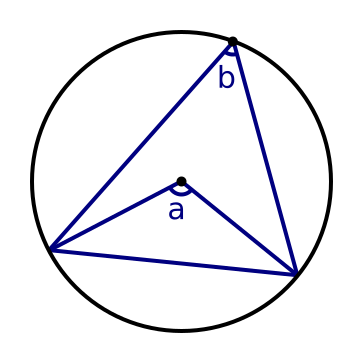# Angle at the centre of a circle is twice the angle at the circumference

Martin McBride, 2020-08-16
Tags circle chord angle
Categories maths gcse geometry

For any chord, we can draw two lines to form an angle at the centre of the circle, angle a.

We can also draw two lines to form an angle at the circumference, b.The angle at the centre of a circle is twice the angle at the circumference.

This theorem is covered in this video on circle theorems: